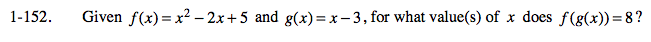### Home > PC > Chapter 1 > Lesson 1.4.2 > Problem1-152

1-152.

Given f(x) = x2 − 2x + 5 and g(x) = x − 3, for what value(s) of x does f(g(x)) = 8? Homework Help ✎Substitute the g function into the f function and set it equal to 8.

f(x – 3) = 8

(x – 3)2 – 2(x – 3) + 5 = 8